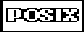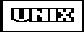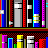times -- Determine Process TimesSYNOPSIS

#include <sys/times.h>

clock_t times(struct tms *buffer);

DESCRIPTION

times stores user and system CPU time for a process and its children. The time information is stored in a tms structure located at the address pointed to by buffer. The tms structure contains the following members:
clock_t tms_utime
is the amount of CPU time used by instructions in the calling process. Under MVS OpenEdition, this does not include time used by instructions in the kernel; however, it does include CPU time for the address space before it became a process.
clock_t tms_stime
is the amount of CPU time used by the system on behalf of the calling process. Under MVS OpenEdition, this value represents kernel time used for the calling process. It does not include time spent calling other system functions on behalf of the calling process.
clock_t tms_cutime
is the total user time for all terminated child processes. This value is calculated by summing the tms_utime and tms_cutime values for all child processes that have terminated.
clock_t tms_cstime
is the total system time for all terminated child processes. This value is calculated by summing the tms_stime and tms_cstime values for all child processes that have terminated.
The clock_t type measures time in clock ticks. The amount of time represented by each clock tick is determined by the CLK_TCK symbol, which is defined in time.h.

RETURN VALUE

If successful, times returns a positive value representing elapsed time, and ((clock_t) -1) if it is unsuccessful. The elapsed time value is referenced from an arbitrary point; hence, it is only useful when making more than one call to times.

Note: OpenEdition computes elapsed time values using fullword (long) arithmetic. If this computation overflows, times indicates an error and sets errno to ERANGE.

EXAMPLE

The following example illustrates the use of times to determine process times:
#include <sys/types.h>
#include <sys/wait.h>
#include <unistd.h>
#include <time.h>
#include <times.h>
#include <stdio.h>
#include <stdlib.h>

int main(void)
{
int i, status;
pid_t pid;
time_t currentTime;
struct tms cpuTime;

if ((pid = fork()) == -1) {         /* Start a child process.   */
perror("fork error");
exit(EXIT_FAILURE);
}
else if (pid == 0) {                /* This is the child.       */
time(&currentTime);
printf("Child process started at %s", ctime(&currentTime));
for (i = 0; i < 5; ++i) {
printf("Counting: %dn", i);  /* count for 5 seconds.     */
sleep(1);
}
time(&currentTime);
printf("Child process ended at %s", ctime(&currentTime));
exit(EXIT_SUCCESS);
}
else {                              /* This is the parent.      */
time(&currentTime);
printf("Parent process started at %s", ctime(&currentTime));

if (wait(&status) == -1 )        /* Wait for child process.  */
perror("wait error");
if (WIFEXITED(status))
printf("Child process ended normally.n");
else
printf("Child process did not end normally.n");

if (times(&cpuTime) < 0)         /* Get process times.       */
perror("times error");
else {
printf("Parent process user time = %fn",
((double) cpuTime.tms_utime)/CLK_TCK);
printf("Parent process system time = %fn",
((double) cpuTime.tms_stime)/CLK_TCK);
printf("Child process user time = %fn",
((double) cpuTime.tms_cutime)/CLK_TCK);
printf("Child process system time = %fn",
((double) cpuTime.tms_cstime)/CLK_TCK);
}
time(&currentTime);
printf("Parent process ended at %s", ctime(&currentTime));
exit(EXIT_SUCCESS);
}
}

RELATED FUNCTIONS

clock